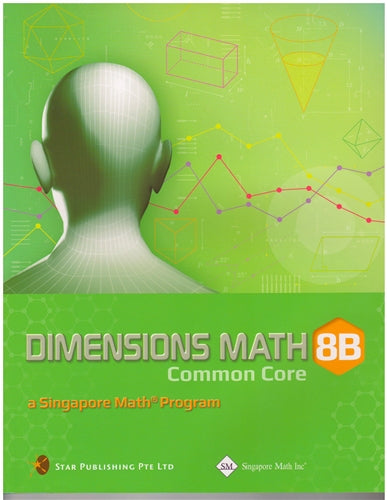# Singapore Math Dimensions Math Textbook 8B

Dimensions Math® Textbook 8B

Chapter 8: Graphs of Linear and Quadratic Functions

8.1 Linear Function

A. Idea of Functions
B. Linear Functions
C. Rate of Change

A. Graph of y = ax2 + bx + c, where a > 0
B. Graph of y = ax2 + bx + c, where a < 0

In a Nutshell
Review Exercise 8

Chapter 9: Graphs in Practical Situations

9.1 Tables, Charts and Graphs
9.2 Distance-Time Graphs

In a Nutshell
Review Exercise 9

Chapter 10: Pythagorean Theorem

10.1 Pythagorean Theorem
10.2 The Converse of Pythagorean Theorem

A. Definition
B. Determination of Right-Angled Triangles

10.3 Applications of Pythagoras Theorem

In a Nutshell
Review Exercise 10

Chapter 11: Coordinate Geometry

11.1 Distance Between Two Points
11.2 Slope of a Straight Line
11.3 Equation of a Straight Line

In a Nutshell
Review Exercise 11

Chapter 12: Mensuration of Pyramids, Cylinders, Cones and Spheres

12.1 Pyramids

A. Introducing Pyramids
B. Nets and Surface Areas of Pyramids
C. Volumes of Pyramids

12.2 Cylinders

A. Volume and Surface Area of a Cylinder

12.3 Cones

A. Introducing Cones
B. Net and Surface Area of a Cone
C. Volume of a Cone

12.4 Spheres

A. Introducing Spheres
B. Volume of a Sphere
C. Surface Area of a Sphere

In a Nutshell
Review Exercise 12

Chapter 13: Data Analysis

13.1 Organizing Data in Frequency Tables
13.2 Bar Graphs and Histograms

A. Bar Graphs
B. Histograms

13.3 Line Graphs and Scatter Plots

A. Line Graphs
B. Scatter Plots

In a Nutshell
Review Exercise 13

14.1 Solving Quadratic Equations by Factorization
14.2 Completing the Square Method
14.4 Graphical Method

In a Nutshell Review Exercise 14

Dimensions Math® G6-8 Textbooks are designed for middle school students. Developed in collaboration between Star Publishing and Singapore Math Inc®, this series follows the Singapore Mathematics Framework and covers the topics in the Common Core State Standards.

The emphasis of this series is on empowering the students to learn mathematics effectively and independently. Depending on the topics covered, different approaches are adopted for the presentation of concepts to facilitate easy understanding by students to internalize concepts and instill in them an interest to explore the topics further.

Each book includes appropriate examples, class activities and diagrams to understand the concepts and apply them. IT skills and values are incorporated as appropriate.

Some features of this series include the following:

1. Class activities which allow the student to learn mathematics through discovery. Some of the class activities make use of Geometer’s Sketchpad (available at keypress.com).
2. Worked examples are followed by a similar question (Try It!) so that students can check if they have understood the concepts presented earlier.
3. An exercise for each lesson including questions in the following sequence:
• Basic Practice (simple questions involving a direct application of the concepts)
• Further Practice (more challenging questions on direct application)
• Maths@Work (questions that apply mathematical concepts to real-life situations)
• Brainworks (questions involving higher order thinking or an open-ended approach to problems)
4. Each chapter is followed by a review exercise; the Extend Your Learning Curve activity encourages students to explore mathematical concepts further or apply mathematics in real-life situations, with some questions requiring sentence or paragraph answers to encourage students to reflect on their learning experiences.
5. The right margins contain remarks on important information, concepts or definitions covered earlier related to the current material to help students recall them, discussion questions, interesting puzzles or facts that are related to mathematics, and some website references.

The answer key at the back of the book provides answers to the Try It! and the problems in the exercises for the Basic PracticeFurther Practice, and Maths@Work questions. It does not include answers to the class activities or the Extend Your Learning Curve activities.